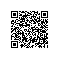# 9种常用的机器学习算法实现

## 简介

1、监督学习（Supervised Learning，SL），这类算法的工作原理是使用带标签的训练数据来学习输入变量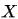转化为输出变量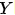的映射函数，换句话说就是求解方程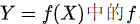。进一步地，监督学习又可细分为如下三类：

• 回归（Regression）：预测一个值，如预测降雨量、房价等，较基础的算法有：Linear Regression
• 分类（Classification）：预测一个标签，如预测“生病”或“健康”，图片上是哪种动物等，较基础的算法有：Logistic Regression、Naive Bayes、K-Nearest Neighbors（KNN）

【另】：集成（Ensembling）也可以归类为监督学习的一种，它将多个单独较弱的机器学习模型的预测结合起来，以产生更准确的预测，较基础的算法有Bagging with Random Forests、Boosting with XGBoost

2、非监督学习（Unsupervised Learning，UL），这类算法的工作原理是从无标签的训练数据中学习数据的底层结构。进一步地，非监督学习又可细分为如下三类：

• 关联（Association）：发现集合中项目同时出现的概率，如通过分析超市购物篮，发现啤酒总是和尿片一起购买（啤酒与尿片的故事），较基础的算法有：Apriori
• 聚类（Clustering）：对数据进行分组，以便组内对象比组间对象更相似，较基础的算法有：K-Means
• 降维（Dimensionality Reduction）：减少数据集的变量数量，同时保证重要的信息不被丢失。降维可以通过特征提取方法和特征选择方法来实现，特征提取是执行从高维空间到低维空间的转换，特征选择是选择原始变量的子集，较基础的算法有：PCA

3、强化学习（Reinforcement Learning，DL），让agent根据当前环境状态，通过学习能够获得最大回报的行为来决定下一步的最佳行为。

## ▐  Linear Regression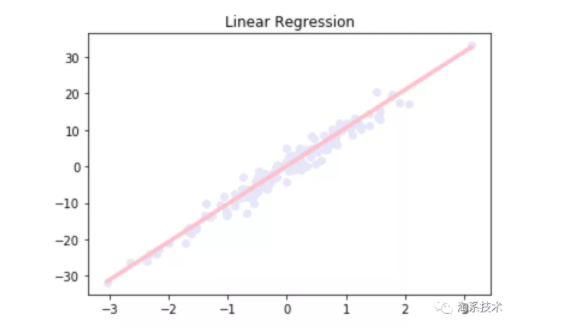## ▐  Logistic Regression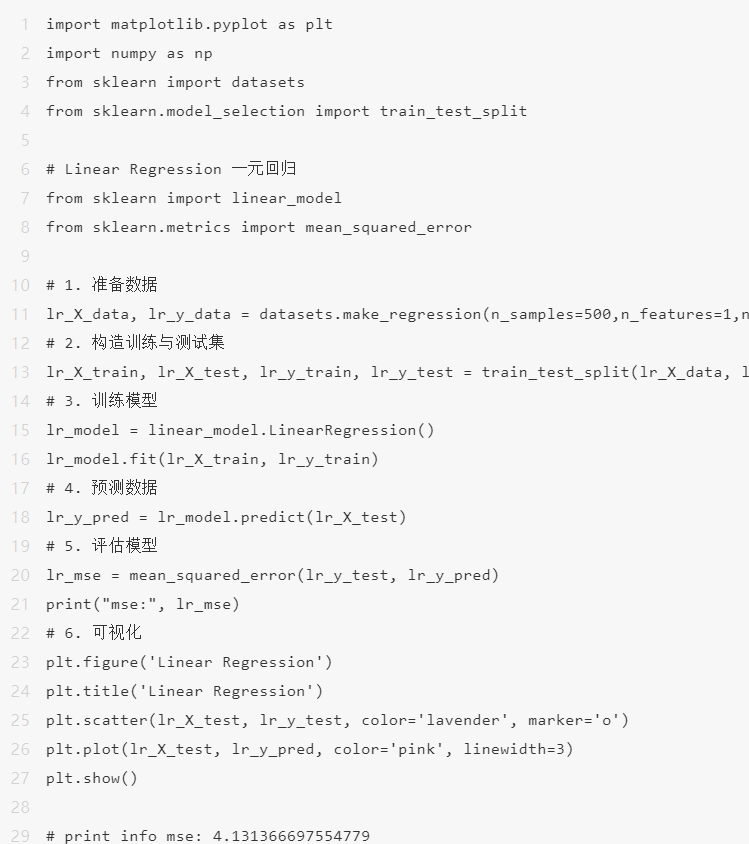## ▐  Naive Bayes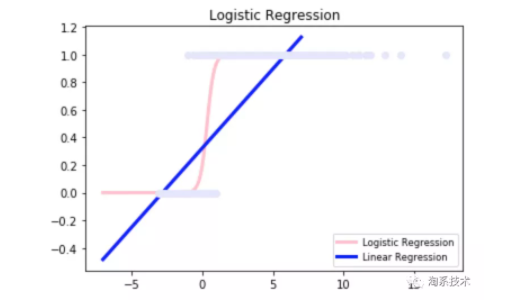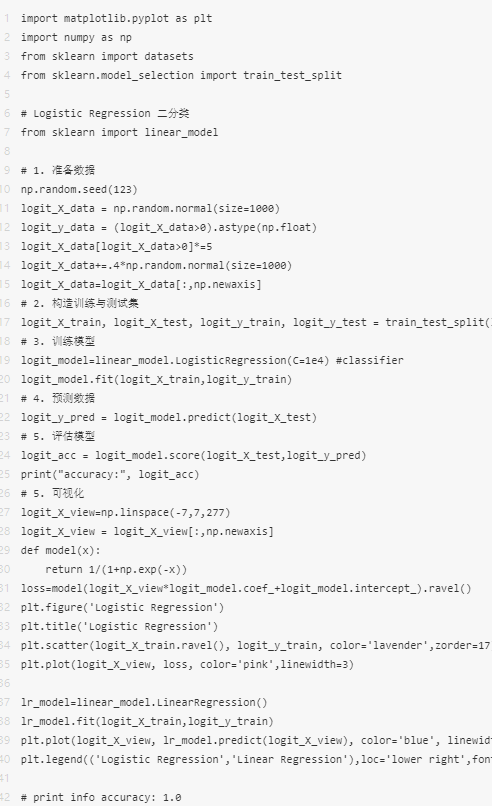## ▐  K-Nearest Neighbors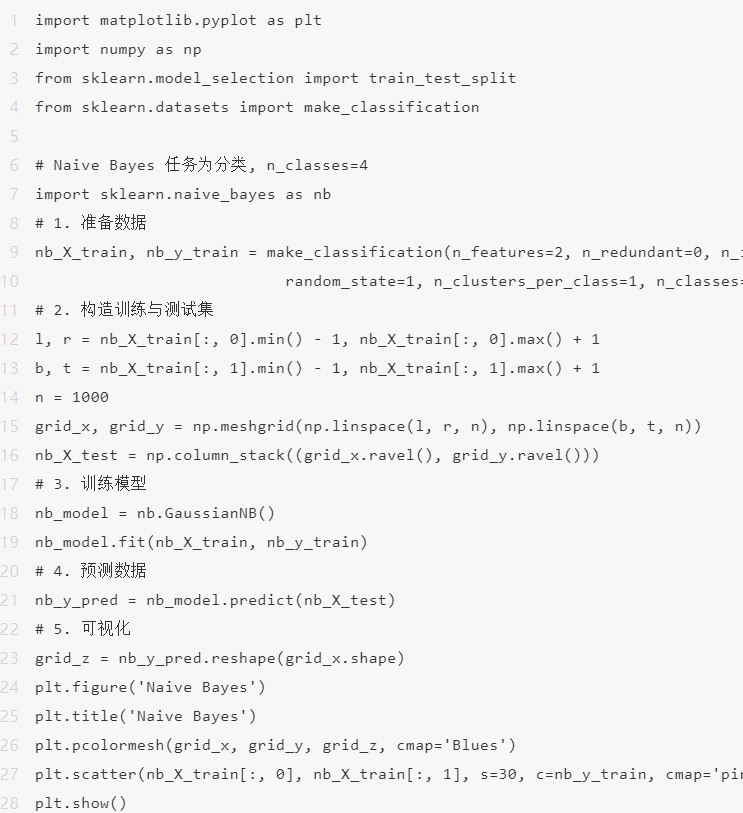## ▐  Decision Tree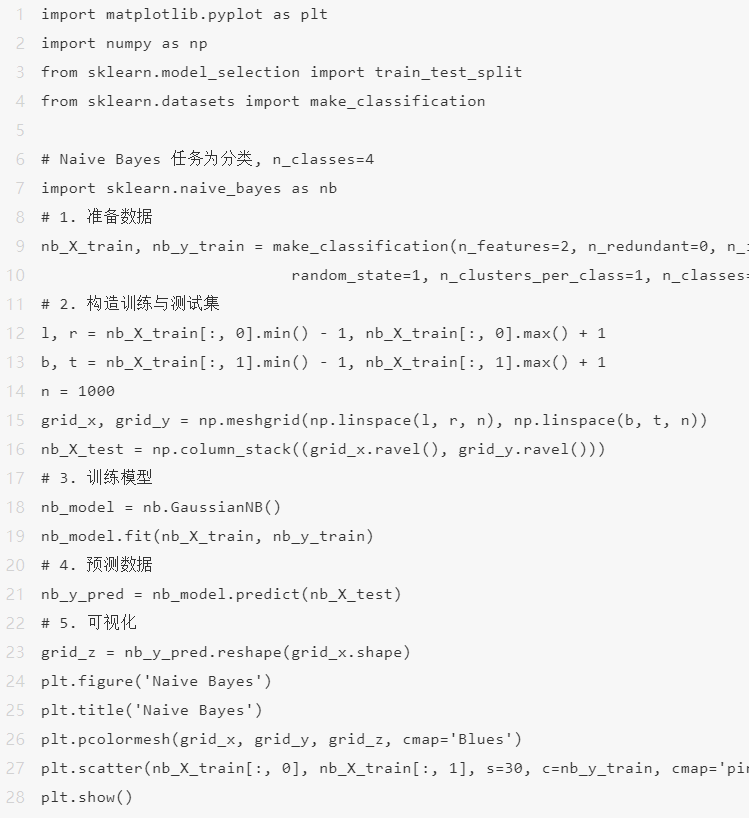## ▐  Random Forest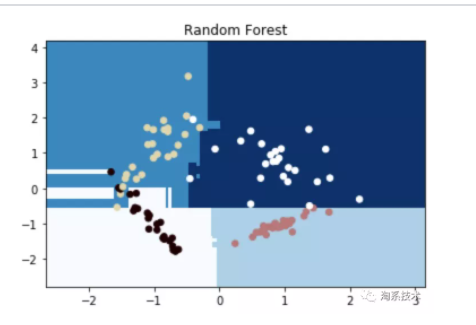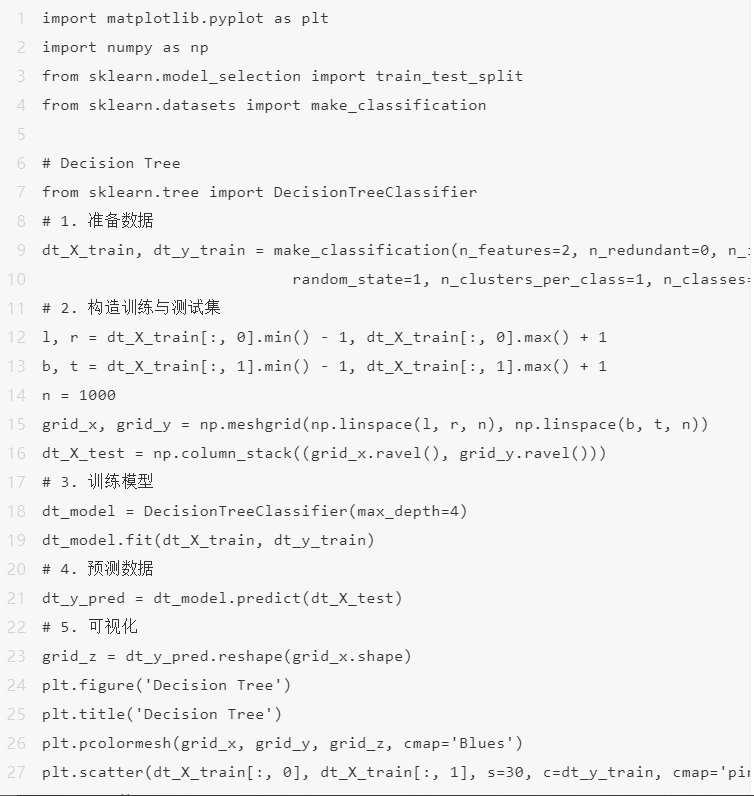## ▐  Support Vector Machines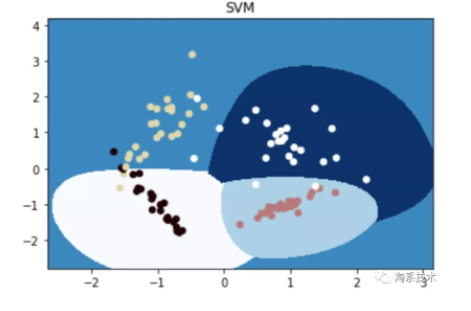import matplotlib.pyplot as pltimport numpy as npfrom sklearn.model_selection import train_test_splitfrom sklearn.datasets import make_classification
# SVMfrom sklearn import svm# 1. 准备数据svm_X_train, svm_y_train = make_classification(n_features=2, n_redundant=0, n_informative=2,                           random_state=1, n_clusters_per_class=1, n_classes=4)# 2. 构造训练与测试集l, r = svm_X_train[:, 0].min() - 1, svm_X_train[:, 0].max() + 1b, t = svm_X_train[:, 1].min() - 1,svm_X_train[:, 1].max() + 1n = 1000grid_x, grid_y = np.meshgrid(np.linspace(l, r, n), np.linspace(b, t, n))svm_X_test = np.column_stack((grid_x.ravel(), grid_y.ravel()))# 3. 训练模型# svm_model = RandomForestClassifier(max_depth=4)svm_model = svm.SVC(kernel='rbf', gamma=1, C=0.0001).fit(svm_X_train, svm_y_train)svm_model.fit(svm_X_train, svm_y_train)# 4. 预测数据svm_y_pred = svm_model.predict(svm_X_test)# 5. 可视化grid_z = svm_y_pred.reshape(grid_x.shape)plt.figure('SVM')plt.title('SVM')plt.pcolormesh(grid_x, grid_y, grid_z, cmap='Blues')plt.scatter(svm_X_train[:, 0], svm_X_train[:, 1], s=30, c=svm_y_train, cmap='pink')plt.show()

## ▐  K-Means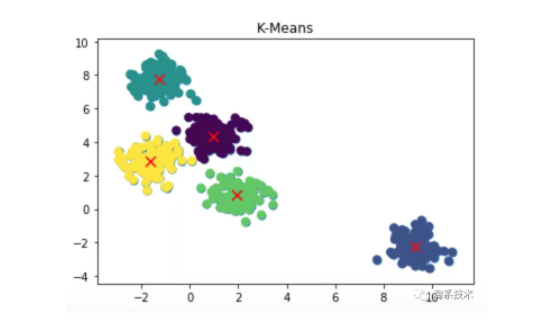import matplotlib.pyplot as pltimport numpy as npfrom sklearn.model_selection import train_test_splitfrom sklearn.datasets.samples_generator import make_blobs
# K-means 任务为聚类 n_classes=5from sklearn.cluster import KMeans
# 1. 准备数据kmeans_X_data, kmeans_y_data = make_blobs(n_samples=500, centers=5, cluster_std=0.60, random_state=0)# 2. 训练模型kmeans_model = KMeans(n_clusters=5)kmeans_model.fit(kmeans_X_data)# 3. 预测模型kmeans_y_pred = kmeans_model.predict(kmeans_X_data)# 4. 可视化plt.figure('K-Means')plt.title('K-Means')plt.scatter(kmeans_X_data[:,0], kmeans_X_data[:, 1], s=50)plt.scatter(kmeans_X_data[:, 0], kmeans_X_data[:, 1], c=kmeans_y_pred, s=50, cmap='viridis')centers = kmeans_model.cluster_centers_plt.scatter(centers[:,0], centers[:, 1], c='red', s=80, marker='x')plt.show()

## ▐  PCA

import matplotlib.pyplot as pltimport numpy as npfrom sklearn.model_selection import train_test_splitfrom sklearn.datasets import make_classification
# PCAfrom sklearn.decomposition import PCAfrom sklearn.datasets import load_iris
# 1. 准备数据pca_data=load_iris()pca_X_data=pca_data.datapca_y_data=pca_data.target# 2. 训练模型, 维度为2pca_model=PCA(n_components=2)  # 3. 降维reduced_X=pca_model.fit_transform(pca_X_data)# 4. 可视化red_x,red_y=[],[]blue_x,blue_y=[],[]green_x,green_y=[],[]
for i in range(len(reduced_X)): if pca_y_data[i] ==0:  red_x.append(reduced_X[i])  red_y.append(reduced_X[i]) elif pca_y_data[i]==1:  blue_x.append(reduced_X[i])  blue_y.append(reduced_X[i]) else:  green_x.append(reduced_X[i])  green_y.append(reduced_X[i])
plt.figure('PCA')plt.title('PCA')plt.scatter(red_x,red_y,c='r')plt.scatter(blue_x,blue_y,c='b')plt.scatter(green_x,green_y,c='g')plt.show()

https://zhuanlan.zhihu.com/p/72513104

https://zhuanlan.zhihu.com/p/139122386

https://www.cnblogs.com/pinard/p/6069267.html

https://www.cnblogs.com/pinard/p/6061661.html

https://zhuanlan.zhihu.com/p/26703300

https://zhuanlan.zhihu.com/p/51165358

https://zhuanlan.zhihu.com/p/49331510

https://zhuanlan.zhihu.com/p/75477709